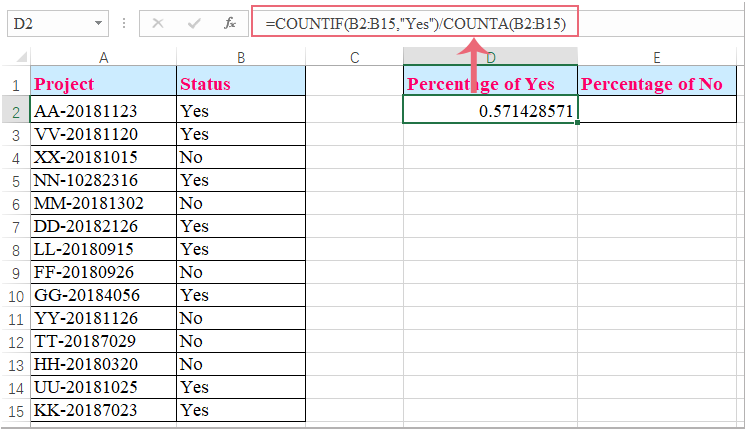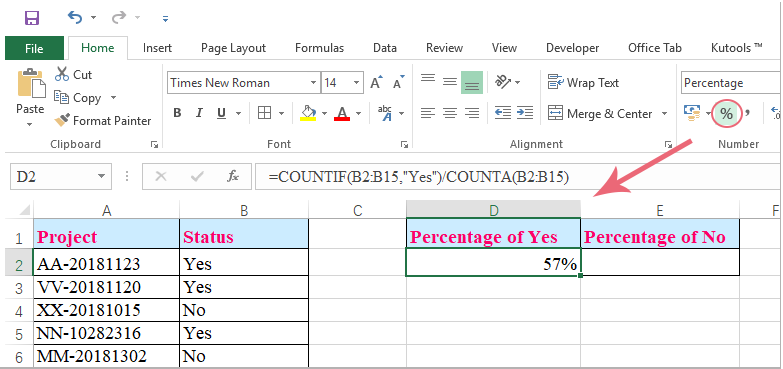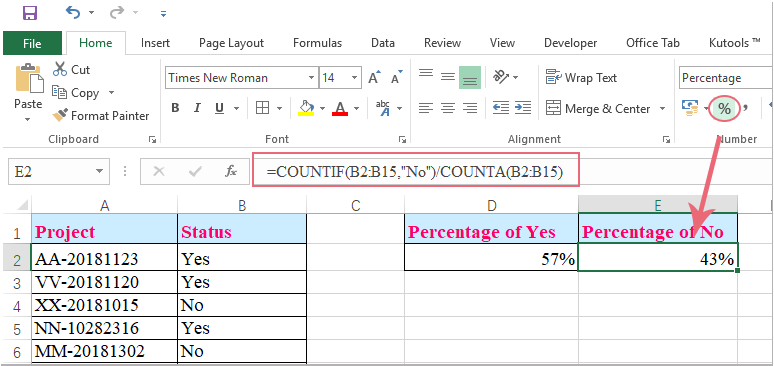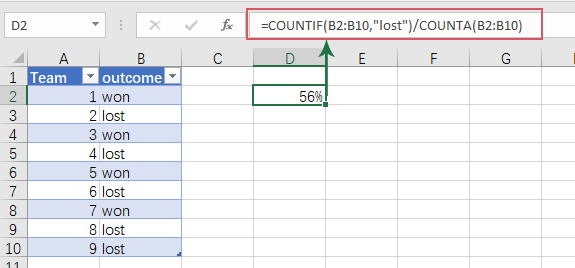## How to calculate the percentage of yes and no from a list in Excel?

How could you calculate the percentage of yes and no text from a list of range cells in Excel worksheet? Maybe this article will help you to deal with the task.

Calculate the percentage of yes and no from a list of cells with formula

#### Calculate the percentage of yes and no from a list of cells with formula

To get the percentage of a specific text from a list of cells, the following formula can help you, please do as this:

1. Enter this formula: =COUNTIF(B2:B15,"Yes")/COUNTA(B2:B15) into a blank cell where you want to get the result, and then press Enter to a decimal number, see screenshot:2. Then you should change this cell format to percent, and you will get the result you need, see screenshot:Notes:

1. In the above formula，B2:B15 is the list of cells which contain the specific text you want to calculate the percentage;

2. To calculate the percentage of no text, please just apply this formula: =COUNTIF(B2:B15,"No")/COUNTA(B2:B15).### Best Office Productivity Tools

Supercharge Your Spreadsheets： Experience Efficiency Like Never Before with Kutools for Excel

 Popular Features: Find/Highlight/Identify Duplicates   |  Delete Blank Rows   |  Combine Columns or Cells without Losing Data   |   Round without Formula ... Super Lookup: Multiple Criteria VLookup  |   Multiple Value VLookup  |   VLookup Across Multiple Sheets   |   Fuzzy Lookup .... Advanced Drop-down List: Quickly Create Drop Down List   |  Dependent Drop Down List   |  Multi-select Drop Down List .... Column Manager: Add a Specific Number of Columns   |   Move Columns   |   Unhide Columns   |   Compare Columns to Select Same & Different Cells ... Featured Features: Grid Focus   |  Design View   |   Big Formula Bar   |  Workbook & Sheet Manager   |  Resource Library (Auto Text)   |  Date Picker   |  Combine Worksheets   |  Encrypt/Decrypt Cells   |  Send Emails by List   |  Super Filter   |   Special Filter (filter bold/italic/strikethrough...) ... Top 15 Toolset:  12 Text Tools (Add Text, Remove Characters, ...)   |   50+ Chart Types (Gantt Chart, ...)   |   40+ Practical Formulas (Calculate age based on birthday, ...)   |   19 Insertion Tools (Insert QR Code, Insert Picture from Path, ...)   |   12 Conversion Tools (Numbers to Words, Currency Conversion, ...)   |   7 Merge & Split Tools (Advanced Combine Rows, Split Cells, ...)   |   Many More...

Kutools for Excel boasts over 300 features, ensuring that what you need is just a click away...

Supports Office/Excel 2007-2021 & newer, including 365   |   Available in 44 languages   |   Enjoy a full-featured 30-day free trial.#### Office Tab Brings Tabbed interface to Office, and Make Your Work Much Easier

• Enable tabbed editing and reading in Word, Excel, PowerPoint, Publisher, Access, Visio and Project.
• Open and create multiple documents in new tabs of the same window, rather than in new windows.
• Increases your productivity by 50%, and reduces hundreds of mouse clicks for you every day!Rated 5 out of 5 · 1 ratings
This comment was minimized by the moderator on the site
I am want to use a function that calculate a rate of 1 to 5 using a percentage of each cell if the answer is yes. Rate = (country x 25%)+(role x 25%) + (age x 25%) + ( risk x 25%)
This comment was minimized by the moderator on the site
Hello,

I am looking to get a percentage of cells populated in a column. I have built a tracking sheet for a project where associates will enter their initials into the cells to show they have completed that task. I would like to show a percentage of tasks completed if that makes sense?

Thanks,
This comment was minimized by the moderator on the site
Hi Mandy,

How do you use this formula =COUNTIF(B2:B15,"Yes")/COUNTA(B2:B15)

But to fetch data across multiple Sheets? For example I am looking for the word 'Yes' in a second sheet and third sheet but would like to populate the result on the first sheet.

Thank
Jas
This comment was minimized by the moderator on the site
Hello, Jas
If you want to get the percentage of Yes from multiple sheets, may be the below formula can help you:

=(COUNTIF(Sheet2!B2:B15,"Yes")+COUNTIF(Sheet3!B2:B15,"Yes"))/(COUNTA(Sheet2!B2:B15)+COUNTA(Sheet3!B2:B15))

But, if you just need to put the result in another sheet, please apply the below formula:
=COUNTIF(Sheet2!B2:B15,"Yes")/COUNTA(Sheet2!B2:B15)

This comment was minimized by the moderator on the site
Team Outcome
1 Won
2 lost
4 lost
5 lost
6 Won

Why does this not work using the Tables parameters ?
This comment was minimized by the moderator on the site
Forgot to mention, this returns 0 no matter the data
This comment was minimized by the moderator on the site
Hello, Dan,
As you said, the formula does not work correctlly in a table format, so, you need to use it in a normal range. Please don't put the formula next to the table, locate it beyond the table, as below screenshot shown:This comment was minimized by the moderator on the site
gracias, me sirvió la formula para calcular el % de SI y NO
Rated 5 out of 5
This comment was minimized by the moderator on the site
How do you use the countif when you are trying 3 different criteria to equal a percentage.
Yes/No/NA
NA- should not impact the total combined percentage of Yes/No Answers. Using as an excel audit tool.

=IF(A23="","",COUNTIF(E23:I23,"Yes")/(COUNTIF(E23:I23,"Yes")+COUNTIF(E23:I23,"NO")))

This formula is still decreasing total score when N/A is selected in cell.
This comment was minimized by the moderator on the site
Hello Brandy,
Thanks for your message. In B1:B10, there are 3 different data: Yes/No/NA. To calculate the percentage of the number of Yes of the total number of Yes and No, please input the formula: =IF(B1="","",COUNTIF(B1:B10,"Yes")/(COUNTIF(B1:B10,"Yes")+COUNTIF(B1:B10,"NO"))). You will get the correct result.
Sincerely,
Mandy
This comment was minimized by the moderator on the site
this worked HOWEVER, when I do a sort the % does not change with the sorted data. How can I get the % to change when I sort?
This comment was minimized by the moderator on the site
Hello Nataile,
Glad to help. When you sort the data, you need to change the range in the Countif formula to absolute. Otherwise, the results will be wrong. For example, the first formula in the artical should be changed to: =COUNTIF(\$B\$2:\$B\$15,"Yes")/COUNTA(B2:B15) . Please have a try.

Sincerely,
Mandy
This comment was minimized by the moderator on the site
Trying to find a way to use the function

=COUNTIF(F3:F17,F21:F35,P3:P17,P21:P35,F39:F53,P39:P53,Z3:Z17,Z21:Z35,Z39:Z53,AJ3:AJ17,AJ21:AJ35,AJ39:AJ53,"No")/COUNTA(F3:F17,F21:F35,P3:P17,P21:P35,F39:F53,P39:P53,Z3:Z17,Z21:Z35,Z39:Z53,AJ3:AJ17,AJ21:AJ35,AJ39:AJ53)
However, it says I can only have 2 arguments. Is there another function I have to use for this many ranges?

Any help is much appreciated!
This comment was minimized by the moderator on the site
Bonjour,

En utilisant votre formule j'arrive a une erreur et rien n'apparait est-ce normal ? d'autant plus que j'ai bien entré la formule ..
Pouvez vous m'aider svp ?
Merci
This comment was minimized by the moderator on the site
you would use "," instead of ":" so your formula would look like [ =COUNTIF(B2,B3,B10,B11,B15,"Yes")/COUNTA(B2,B3,B10,B11,B15) ]
This comment was minimized by the moderator on the site
I need a formula that functions similar to [ =COUNTIF(B2:B15,"Yes")/COUNTA(B2:B15) ] but that is for specific cells not a range. How would that work? if only some of them need to be incuded like B3,B3, B 10, B11, B15. How would that change the formula?
This comment was minimized by the moderator on the site
you would use "," instead of ":" so your formula would look like [ =COUNTIF(B2,B3,B10,B11,B15,"Yes")/COUNTA(B2,B3,B10,B11,B15) ]
This comment was minimized by the moderator on the site
I have the same question and can't find the answer...did you have any success?
There are no comments posted here yet